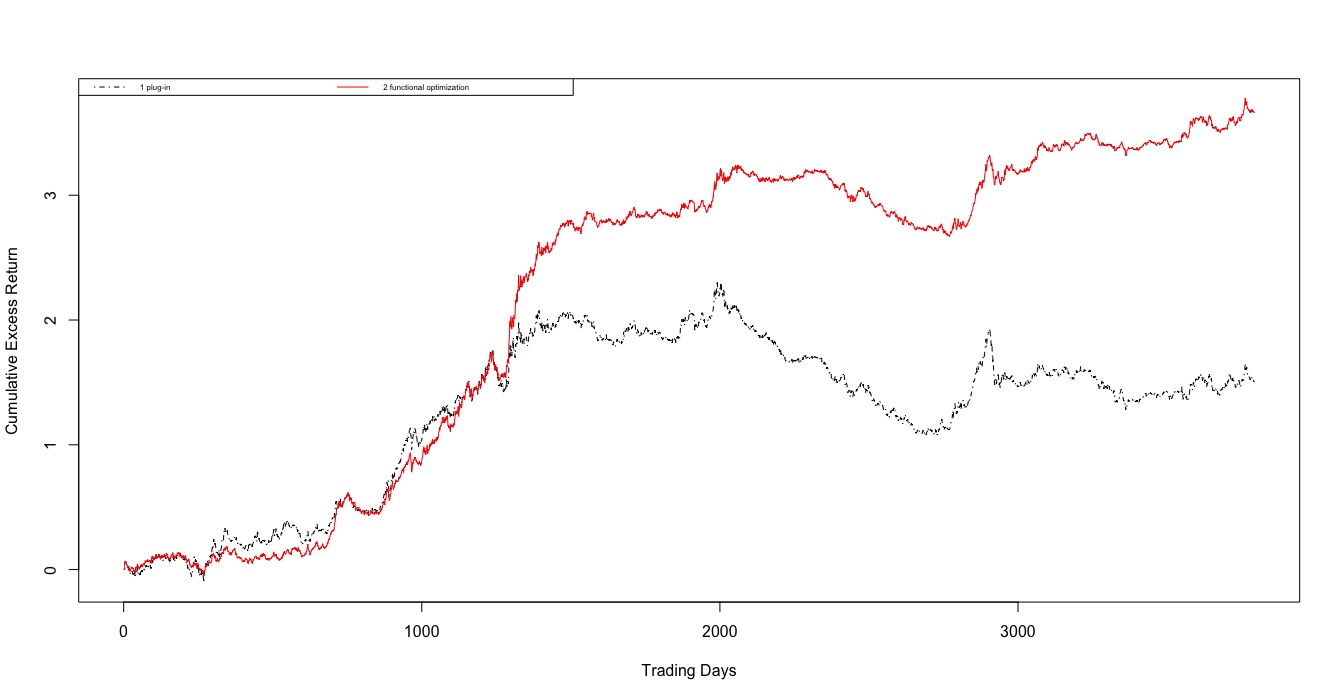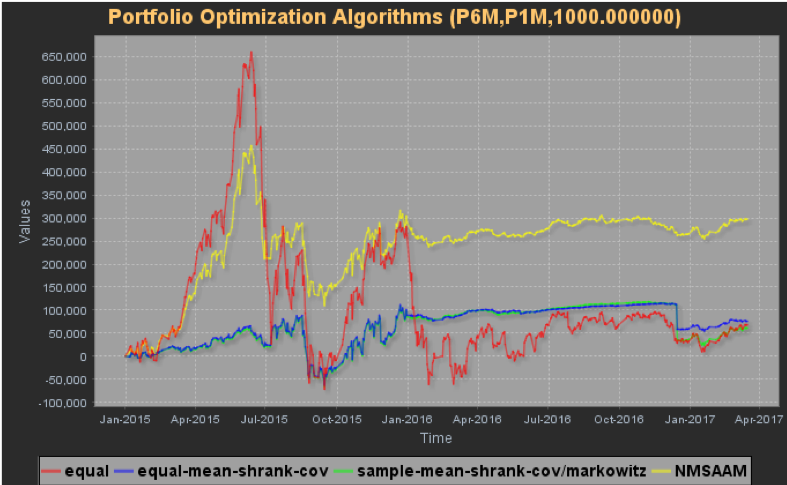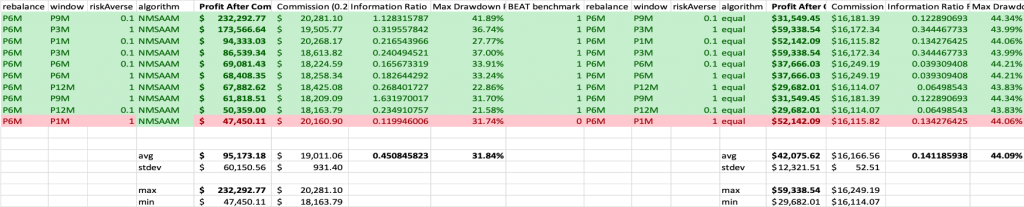## NM Stochastic Asset Allocation Model (NMSAAM)

Invented by NM FinTech, NM Stochastic Asset Allocation Model (NMSAAM) is one of the most advanced and effective asset allocation models in the market. It is a radically different approach than most theories developed in the last 60 years and effectively fixed most of the existing problems within the industry. This model enables us to solve root cause for model failure and to fix the existing shortcomings and limitations of Markowitz Model including the difficulties in estimating expected returns and  covariances matrix.## Markowitz Modern Portfolio Theory

In 1952, Harry Markowitz presented an essay on “Modern Portfolio Theory” for which he received a Noble Price in Economics. His findings greatly changed the asset management industry, and his theory is still considered as cutting edge in portfolio management.

There are two main concepts in Markowitz Modern Portfolio Theory, which are;

• Any investor’s goal is to maximize Return for any level of Risk

• Risk can be reduced by creating a diversified portfolio of unrelated assets

Markowitz model is foundational to Modern portfolio theory. It is a portfolio optimization model which assists in the selection of the most efficient portfolio by analyzing various possible portfolios of the given securities. By choosing securities that do not ‘move’ exactly together, the model shows investors how to reduce their risk. That’s why the Markowitz model is also called Mean-Variance model due to the fact that it is based on expected returns (mean) and the standard deviation (variance) of the various portfolios.

## Limitations Of Markowitz Model

The limitations of Markowitz Model is that it cannot well estimate for expected return and covariance matrix.

Cause for Model Failure: require the knowledge of expected returns and covariances.

–Estimation of covariances may be possible

–Estimation of expected returns is probably the single most challenging problem in finance

## Difficulties In Estimating Expected Returns And Covariances Matrix

To accurately estimate the means and covariances needed, it would take 250 years of data for 25 assets, 500 years for 50 assets

• CSI has 300, 500 or 800 stocks
• not even considering the time varying properties of these parameters

## Our Solutions

NMSAAM can fundamentally improve the problems mentioned above by assuming that expected returns and covariances are not available and then set them as random which is a radically different approach than most theories developed in the last 60 years.

$$\ Max$$ {$$\ {E(w’r_{n+1})-\lambda Var({w’r_{n+1})}}$$}

• $$\ w′ r_{n+1}$$ is random
• We can add in other constraints, e.g., long-only, cardinality

The fundamental innovation is that we assume expected returns and covariances are not available, therefore they are random.

In addition, we have effective ways to estimate the distributions of expected returns and covariances

## Example of a fund allocation in ChinaCumulative Return
lambda
Markowitz Method
Functional Approach
SR
excess return (benchmark:SP500)
lb=0
1.476462
3.642051
lb=-0.02
1.53067
3.940823
lb=-1
3.860854
8.317082
return
lb=0
1.500063
2.374880
lb=-0.02
1.510211
2.262659
MV
z_0.9
excess return (benchmark: SP500)
lb=0
1.569794
5.298314
10
equally weighted approach
functional approach
SR
excess return (Benchmark: SP500)
-0.2052926
2.1244294
return
0.9092214
2.2204301

## Empirical Results• Using a pool of ETFs provided by a bank in China, we compare the asset allocation results of NMSAAM to 1/N (and other models).
• Our results show that NMSAAM out performs 1/N in a wide range of parameters, hence stability.
• NMSAAM properties:
• When the market rallies big, it rides the trend but not to the extreme extend, giving good and risk-controlled profits
• When the market crashes, it limits the drawdown
• When the market walks sideway (since 2016/1), it has a much smaller volatility and smoother pnl curve

## Best Asset Allocation TheoryFrom the table, we see that for {rebalance = 6M}, almost all (except 1) parameter sets out perform 1/N

• The average PnL is 2.26x of that of equal-weighting
• The max profit is 3.91x, the min profit 1.6x
• The Sharpe ratio is 0.45 vs. 0.14

We repeat the analysis for the whole (reasonable) parameter space

• NMSAAM out performs 1/N 55.56% in terms of final PnL
• Average PnL $64,265 vs.$53,052, max drawdown 27.72% vs 42.79%， Sharpe ratio 0.26 vs. 0.21

Conclusion: NMSAAM consistently out performs equal-weighting and is the probably the best asset allocation theory to date.

## Applications

• ### Indices

Alternatives to SPX, CSI, HSI, FTSE, Euronext, DAX, NIKKEI, Nifty with higher Sharpe ratio and lower volatility

• ### Partial Indices

Adding a cardinality constraint will enable good enough tracking of an index using only a subset of the stocks